LIMITED PROMO

Integrated logs, metrics and traces for faster troubleshooting Get offer

Back to blog results

October 22, 2015

# Rapid Similarity Search with Weighted Min-Hash

We are in the era of big data characterized by data sets that are too large, fast and complicated to be handled by standard methods or tools. An example of such kind of big data sets is machine-generated logs. At Sumo Logic, our analytics team has been focused on studying new techniques for summarizing, analyzing and mining machine-generated logs. Our production service is processing hundreds of terabytes of machine-generated logs per day. One technique contributing for such huge scalability is the min-hash, which is an instance of locality sensitive hashing.

### Why min-hash?

Let us consider the problem of finding similar machine logs: provided $$n$$ logs, the goal is to build a data structure that, given a query log $$q$$, return logs that are similar to the query. Suppose each log is represented by a set of tokens and Jaccard index is used to measuring their pairwise similarity as: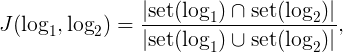where $$J \in [0, 1]$$, $$\mbox{log}_1$$ and $$\mbox{log}_2$$ are two machine logs, and $$\mbox{set}(.)$$ is the function used to tokenizing a machine log into a set of tokens, a straightforward approach for finding similar logs to the query $$q$$ is to iterate all $$n$$ logs and compute their Jaccard similarities to $$q$$. The time consumption of this approach is $$O(n)$$, which is unacceptable for very large datasets of high-dimensional items. The min-hash technique is a much faster solution to the above problem of finding similar logs and its time consumption can be close to $$O(1)$$ . The basic idea is to design a hashing trick that maps similar machine logs into the same bucket so that only logs within the same bucket need to be considered more closely. Let $$h$$ denote a hashing function that maps a token to a random integer: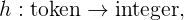then the min-hash function will map $$\mbox{log}$$ into the following bucket: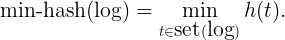We can verify that the probability of two machine logs being mapped to the same bucket is equal to their Jaccard similarity, that is: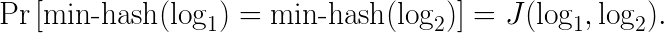The min-hash technique has been widely used in industries such as news recommendation, near duplicate web page detection and image search. At Sumo Logic, our analytics team is adopting it for matching machine logs to signatures and also for inferring new signatures from machine logs.

### Considering token weights

In min-hash, all tokens are considered to have equal weights, and therefore have equal probabilities to be selected as the token with the minimal hashing value. However in reality, we wish to treat tokens as having different weights, in particular in applications such as document retrieval, text mining and web search. A famous approach to weighting tokens in document retrieval is the inverse document frequency (idf). Let $$t$$ be a token, then idf weights $$t$$ as: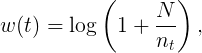where $$n_{t}$$ is the total frequency of $$t$$ appearing in all documents and $$N$$ is the total number of documents. The idf approach puts small weights on frequent tokens and large weights on rare tokens. This unequal assignment of token weights will decrease effects of common tokens and let more informative tokens pop out, thus leading to significant improvement on accuracy and relevance of retrieved results.

Like documents, machine logs consist of tokens, which we may wish to weigh differently. For example, in the machine log:

INFO [hostId=monitor-1] [module=GLASS] [localUserName=glass] [logger=LeaderElection] [thread=Thread-2] Updating leader election; Found 18 elections to update",

bold tokens are more informative than others and thus we hope them have larger weights. It can also be useful to incorporate token weights when measuring the similarity between two logs, which in fact leads to the weighted Jaccard approach defined as: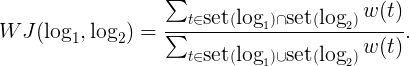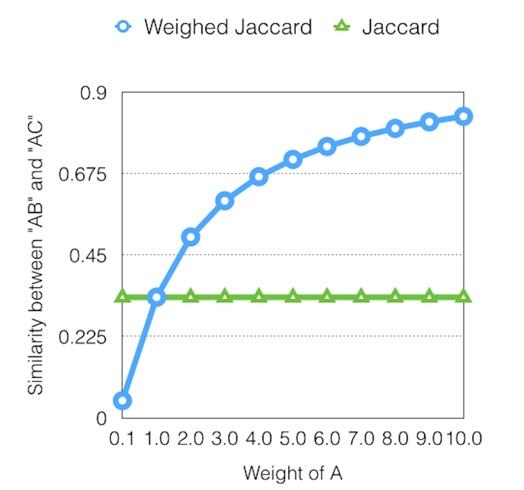The weighted Jaccard similarity is a natural generalization of Jaccard similarity. It will become Jaccard similarity if all token weights $$w(t)$$ are set as $$1.0$$. The figure above gives an example of showing Jaccard and weighted Jaccard similarities between two synthetic machine logs “A B” and “A C” in which weights of tokens “B” and “C” are fixed as $$1.0$$, while the weight of token “A” is changing from $$0.1$$ to $$10$$. It tells us that incorporating token weights can have a major impact on the computed similarity.

### Weighted min-hash

Suppose tokens have different weights and these weights are also known beforehand, the question now becomes how to incorporate token weights into min-hash so that similar logs under weighted Jaccard similarities have high probabilities to be mapped into the same bucket. Note in min-hash, the hashing function, which is a mapping from a token to a random integer, is only used for perturbing orders of tokens. That means you can use original tokens as hashing keys. You can also use randomly-generated strings as hashing keys. The advantage of randomly-generated keys is that you can assign different numbers of random keys to the same token according to its weight. In particular, tokens with large weights should be assigned more random keys, while tokens with small weights should be assigned less random keys. That is the core trick of weighted min-hash. If all token weights are assumed to be positive integers which can be realized by scaling and discretization, then a simple approach is to generate $$w(t)$$ random keys for the token $$t$$ where $$w(t)$$ is its weight. For example, if the weight of token “fail” is $$3$$, then we will assign $$3$$ random keys such as “fail0″, “fail1″, “fail2″ to it.

The weighted min-hash runs min-hash on randomly-generated keys, instead of original keys. The weighted min-hash function becomes: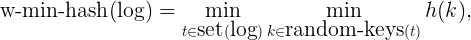where $$\mbox{random-keys}(t)$$ is the set of randomly-generated keys for the token $$t$$ according to its weight. We can verify that for integer-valued weights:Apart from the random keys method described above, monotonic transformation is another approach for incorporating token weights into min-hash. In, the authors did a lot of experiments to compare min-hash and weighed min-hash for image retrieval and concluded that weighted min-hash performs much better than min-hash. Our analytics team got the same conclusion after running min-hash and weighted min-hash for machine log analysis.

 A. Rajaraman and J. Ullman, Mining of Massive Datasets, Cambridge University Press, 2012.

 K. Jones, A Statistical Interpretation of Term Specificity and Its Application in Retrieval, Journal of Documentation, pp. 11-21, 1972

 O. Chum, J. Philbin, and A. Zisserman, Near Duplicate Image Detection: Min-Hash and Tf-Idf Weighting, BMCV, pp. 493-502, 2008

 A. Das, M. Datar, and A. Garg, Google News Personalization: Scalable Online Collaborative Filtering, WWW, pp. 271-280, 2007

 O. Chum, M. Perdoch, and J. Matas, Geometric min-Hashing: Finding a (Thick) Needle in a Haystack, CVPR, pp. 17-24, 2009

 S. Ioffe, Improved Consistent Sampling, Weighted MinHash and L1 Sketching, ICDM, pp.246-255, 2010

### Complete visibility for DevSecOps

Reduce downtime and move from reactive to proactive monitoring.

#### Sumo Logic Continuous Intelligence Platform™

Build, run, and secure modern applications and cloud infrastructures.